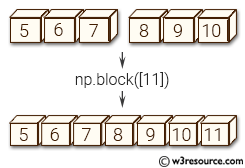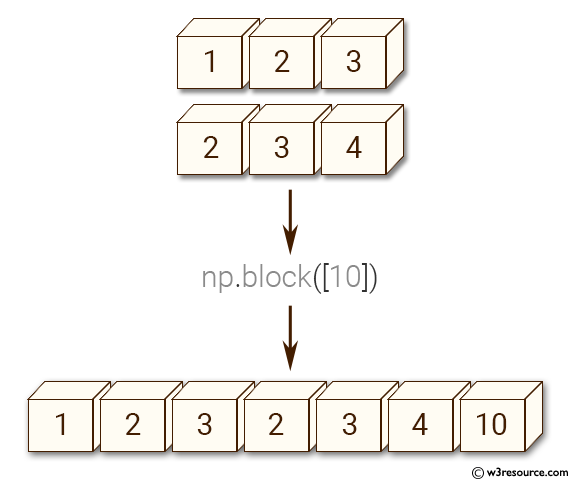# NumPy Array manipulation: block() function

## numpy.block() function

The block() function assembles an nd-array from nested lists of blocks.
Blocks in the innermost lists are concatenated along the last dimension (-1), then these are concatenated along the second-last dimension (-2), and so on until the outermost list is reached.
Blocks can be of any dimension, but will not be broadcasted using the normal rules. Instead, leading axes of size 1 are inserted, to make block.ndim the same for all blocks. This is primarily useful for working with scalars, and means that code like np.block([v, 1]) is valid, where v.ndim == 1.
When the nested list is two levels deep, this allows block matrices to be constructed from their components.

Syntax:

`numpy.block(arrays)`Version: 1.15.0

Parameter:

Name Description Required /
Optional
arrays If passed a single ndarray or scalar (a nested list of depth 0), this is returned unmodified (and not copied).
Elements shapes must match along the appropriate axes (without broadcasting), but leading 1s will be prepended to the shape as necessary to make the dimensions match.
Required

Return value:

block_array : ndarray The array assembled from the given blocks.
The dimensionality of the output is equal to the greatest of: * the dimensionality of all the inputs * the depth to which the input list is nested

Raises: ValueError -

• If list depths are mismatched - for instance, [[a, b], c] is illegal, and should be spelt [[a, b], [c]]
• If lists are empty - for instance, [[a, b], []]

Example-1: numpy.block()

``````import numpy as np
>>> A = np.eye(2) * 2
>>> B = np.eye(3) * 3
>>> np.block([
...     [A,               np.zeros((2, 3))],
...     [np.ones((3, 2)), B               ]
... ])
array([[ 2.,  0.,  0.,  0.,  0.],
[ 0.,  2.,  0.,  0.,  0.],
[ 1.,  1.,  3.,  0.,  0.],
[ 1.,  1.,  0.,  3.,  0.],
[ 1.,  1.,  0.,  0.,  3.]])
``````

Example-2: numpy.block()

``````import numpy as np

>>> np.block([1, 2, 3])
array([1, 2, 3])
>>> a = np.array([1, 2, 3])
>>> b = np.array([2, 3, 4])
>>> np.block([a, b, 10])
array([1, 2, 3, 2, 3, 4, 10])
``````

Pictorial Presentation:Python - NumPy Code Editor:

Previous: vstack()
Next: Splitting arrays split()

﻿